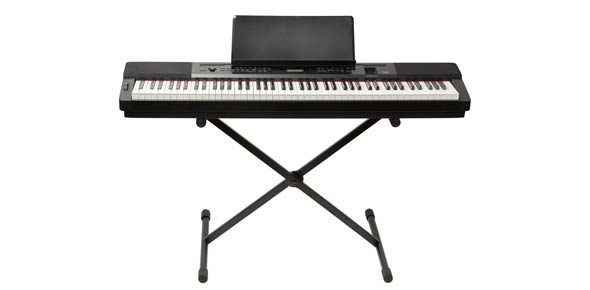# Piano Quiz 1

27 Questions | Total Attempts: 162SettingsBasic piano knowledge Dynamics, rhythm, time signatures, finger numbers, and more.

• 1.
Keys to the left end of the keyboard sound _______ .
• A.

High

• B.

Low

• 2.
Keys to the Right end of the keyboard sound _______ .
• A.

High

• B.

Low

• 3.
How many beats does a quarter note get?
• A.

1

• B.

2

• C.

3

• D.

4

• 4.
How many beats does a half note get?
• A.

1

• B.

2

• C.

3

• D.

4

• 5.
How many beats does a whole note get?
• A.

1

• B.

2

• C.

3

• D.

4

• 6.
You thumbs are your ______ .
• A.

1 fingers

• B.

2 fingers

• C.

3 fingers

• D.

4 fingers

• E.

5 fingers

• 7.
You pinkys are your ______ .
• A.

1 fingers

• B.

2 fingers

• C.

3 fingers

• D.

4 fingers

• E.

5 fingers

• 8.
means to play ________ .
• A.

Soft

• B.

Moderately loud

• C.

Loud

• 9.
means to play ________ .
• A.

Soft

• B.

Moderately loud

• C.

Loud

• 10.
means to play ________ .
• A.

Soft

• B.

Moderately loud

• C.

Loud

• 11.
stands for ________ .
• A.

Piano

• B.

Mezzo forte

• C.

Forte

• 12.
stands for ________ .
• A.

Piano

• B.

Mezzo forte

• C.

Forte

• 13.
stands for ________ .
• A.

Piano

• B.

Mezzo forte

• C.

Forte

• 14.
Dynamics
• A.

How loud or soft to play

• B.

The number of beats in each note

• 15.
This is a ________ .
• A.

Time signature

• B.

Whole note

• C.

Treble clef

• D.

Quarter note

• E.

Bass clef

• F.

Half note

• G.

Dynamic sign

• 16.
The top number in a time signature tells us ______________________ .
• A.

The number of beats in each measure

• B.

What kind of note gets a beat

• C.

How many times to repeat the song

• 17.
The bottom number in a time signature tells us ______________________ .
• A.

The number of beats in each measure

• B.

What kind of note gets a beat

• C.

How many times to repeat the song

• 18.
This is a ________ .
• A.

Time signature

• B.

Whole note

• C.

Treble clef

• D.

Quarter note

• E.

Bass clef

• F.

Half note

• G.

Dynamic sign

• H.

Double bar line

• I.

Repeat sign

• 19.
This is a ________ .
• A.

Time signature

• B.

Whole note

• C.

Treble clef

• D.

Quarter note

• E.

Bass clef

• F.

Half note

• G.

Dynamic sign

• H.

Double bar line

• I.

Repeat sign

• 20.
This is a ________ .
• A.

Time signature

• B.

Whole note

• C.

Treble clef

• D.

Quarter note

• E.

Bass clef

• F.

Half note

• G.

Dynamic sign

• H.

Double bar line

• I.

Repeat sign

• 21.
This is a ________ .
• A.

Time signature

• B.

Whole note

• C.

Treble clef

• D.

Quarter note

• E.

Bass clef

• F.

Half note

• G.

Dynamic sign

• H.

Double bar line

• I.

Repeat sign

• 22.
This is a ________ .
• A.

Time signature

• B.

Whole note

• C.

Treble clef

• D.

Quarter note

• E.

Bass clef

• F.

Half note

• G.

Dynamic sign

• H.

Double bar line

• I.

Repeat Sign

• 23.
This is a ________ .
• A.

Time signature

• B.

Whole note

• C.

Treble clef

• D.

Quarter note

• E.

Bass clef

• F.

Half note

• G.

Dynamic sign

• H.

Double bar line

• I.

Repeat sign

• 24.
This is a ________ .
• A.

Time signature

• B.

Whole note

• C.

Treble clef

• D.

Quarter note

• E.

Bass clef

• F.

Half note

• G.

Dynamic sign

• H.

Double bar line

• I.

Repeat sign

• 25.
This is a ________ .
• A.

Time signature

• B.

Whole note

• C.

Treble clef

• D.

Quarter note

• E.

Bass clef

• F.

Half note

• G.

Dynamic sign

• H.

Double bar line

• I.

Repeat sign

Related Topics